# memnet

#### 2018-10-31

The `memnet` package provides efficient implementations of network science tools to facilitate research into human (semantic) memory. In its current version, the package contains several methods to infer networks from verbal fluency data, various network growth models, diverse (switcher-) random walk processes, and tools to analyze and visualize networks.

The majority of `memnet` is written in C++ to deliver maximum performance.

Have questions, found annoying errors, or have need/recommendation for additional functionality? Please don’t hesitate to write me at `dirk.wulff@gmail.com` or `https://github.com/dwulff/memnet`. Thanks!

## Networks from verbal fluency data

Verbal fluency is a commonly employed task in cognitive science and neuropsychological diagnosis that requires individuals to retrieve within a limited time window, e.g., 1 minute, as many elements from a natural category as they can. Typically, researchers interpret the sequences that individuals produce in this task as reflecting the underlying semantic network structure. Several methods have been proposed to infer the network structure from verbal fluency including the three methods implement here: `community_graph`, `threshold_graph`, and `rw_graph`. Of these three, `community_graph` is the most complex. It adds edges for each pair of produced elements that occur within a window of `l` steps and then retains only those that occur more often than `min_cooc` and what is expected by chance giving a false positive rate of `crit`. `threshold_graph` and `rw_graph` are both nested within `community_graph`. The former retains `min_cooc` as a parameter but sets `l = 1` and `crit = 1`, implying that only adjacent nodes are considered and that chance expectations are not directly taken into account. The latter sets all parameters to 1 by including edges for every adjacent pair of nodes. The results are illustrated below.

``````library(memnet)

# get data
data("animal_fluency")
age = as.numeric(names(animal_fluency))

# infer networks for age > 70
net_comunity = community_graph(animal_fluency[age > 70])
net_threshold = threshold_graph(animal_fluency[age > 70])
net_rw = rw_graph(animal_fluency[age > 70])

# show stats
#>         |V|         |E|           k           C          Cc           L
#>  91.0000000 170.0000000   1.8681319   0.2890767  14.0814358   3.6721981
#>          Lc           S           A           p
#>   4.1809481   3.3680006  -0.2343542   0.9780220
#>          |V|          |E|            k            C           Cc
#>  70.00000000 152.00000000   2.17142857   0.31567992  10.17652372
#>            L           Lc            S            A            p
#>   3.07778295   3.63950362   2.79612958   0.06264176   0.94285714
#>         |V|         |E|           k           C          Cc           L
#> 209.0000000 747.0000000   3.5741627   0.1788195  10.4565117   3.0591645
#>          Lc           S           A           p
#>   3.7885372   2.7600393   0.0781339   1.0000000

# plot
network_plot(edg_to_adj(net_comunity), nod_cex = 2, lab_cex = 1)``````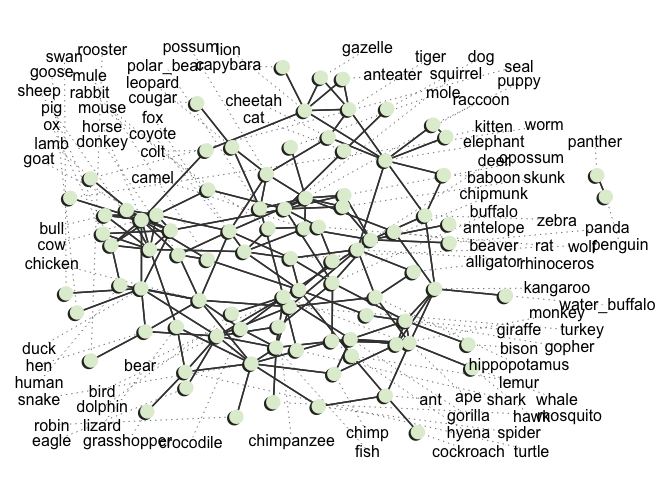``network_plot(edg_to_adj(net_threshold), nod_cex = 2, lab_cex = 1)``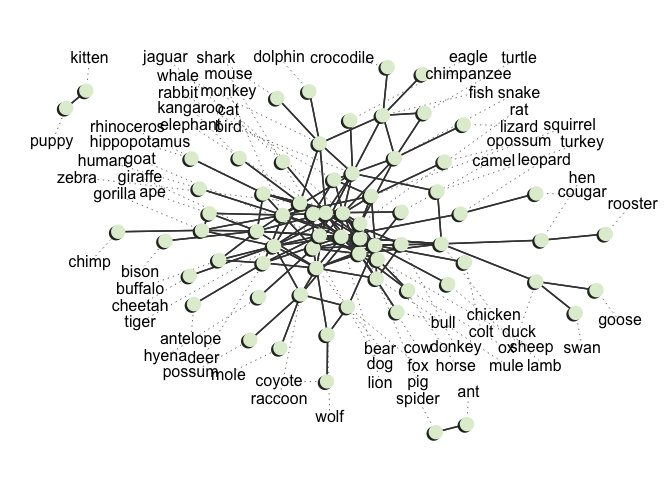``network_plot(edg_to_adj(net_rw), nod_cex = 2, lab_cex = .5, lab_lwd = 1, lab_grid_size = 70)``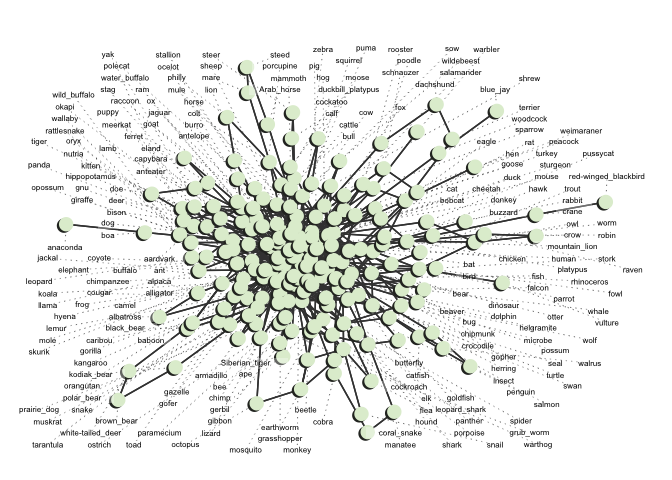``````
# inspect neighborhood of cat
neighborhood_plot(edg_to_adj(net_comunity), k = 3, node = 'cat', nod_cex = 2, lab_cex = 1)``````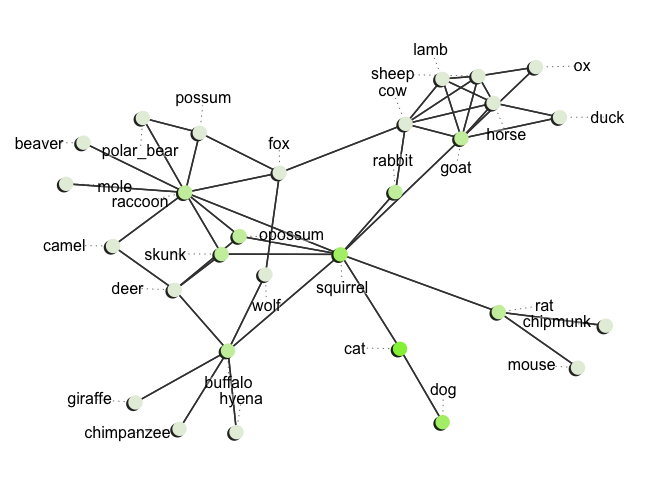``neighborhood_plot(edg_to_adj(net_threshold), k = 3, node = 'cat', nod_cex = 2, lab_cex = 1)``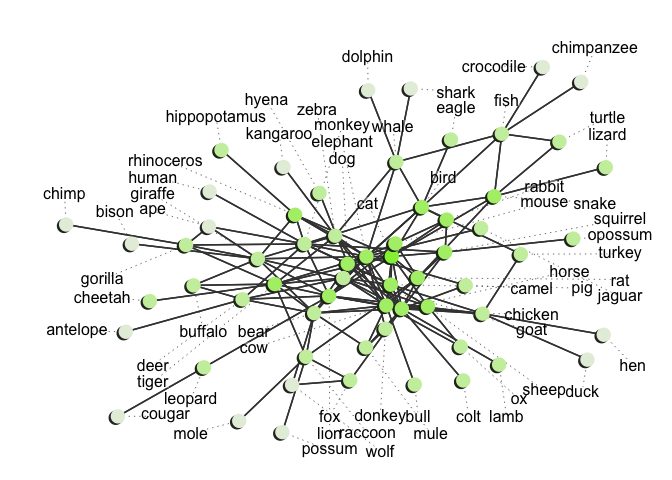``neighborhood_plot(edg_to_adj(net_rw), k = 3, node = 'cat', nod_cex = 2, lab_cex = 1)``